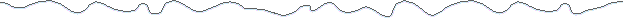On-Axis Field

of an Ideal Helmholtz CoilThese simple formulas use the formula for field due to a current loop to obtain the magnetic field at any point along the axis of an ideal Helmholtz coil.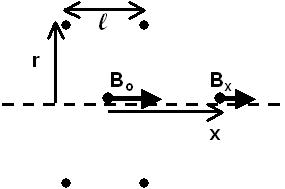Ideal Helmholtz coil in cross section view.

The ideal Helmholtz coil consists of two coaxial circular current loops with the same radius, separated from each other by one radius. In other words, the loops are l apart, such that l = r.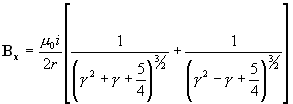Bx is the magnetic field, in teslas, at any point on the axis of the Helmholtz coil. The direction of the field is perpendicular to the plane of the loops.

m 0 is the permeability constant (1.26x10-6 H/m)

i is the current in the wire, in amperes.

r is the radius of the current loops, in meters.

g is the ratio, x/r, where x is the distance, on axis, from the center of the Helmholtz coil, and r is the radius of the coil.

Note: different units for r may be used as long as the permeability constant is correct for that unit system.Special Case: x = 0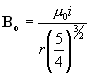Bo is the magnetic field, in teslas, at the center of the Helmholtz coil. The direction of the field is perpendicular to the plane of the loops.The following graph demonstrates the superior central field uniformity of an ideal Helmholtz coil when compared to a solenoid of the same aspect ratio (that is, a solenoid which is as long as its radius):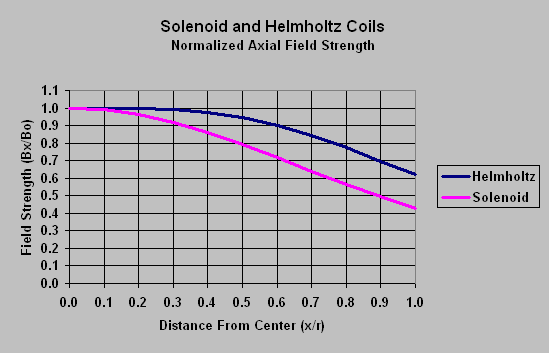Magnet Formulas web site, © 2005 by Eric Dennison. I am developing an updated and expanded version of the magnet web site and encourage you to visit this work in progress and comment on what you see. As a self-employed software engineer trying to balance time between work and magnets, your encouragement in building this site is always welcome! Please consider clicking the PayPal link and making a contribution to support the maintenance and development of this site. Thank you for visiting!